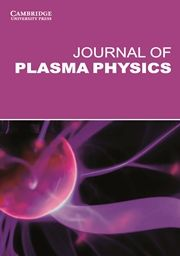Axisymmetric reconnecting plasmoids are secondary magnetic islands, which are formed due to plasmoid instability. At high Lundquist number, the elongated current sheet becomes MHD unstable due to the plasmoid instability (Biskamp Reference Biskamp 1986; Tajima & Shibata Reference Tajima and Shibata 1997; Loureiro, Schekochihin & Cowley Reference Loureiro, Schekochihin and Cowley 2007; Bhattacharjee et al. Reference Bhattacharjee, Huang, Yang and Rogers 2009; Daughton et al. Reference Daughton, Roytershteyn, Albright, Karimabadi, Yin and Bowers 2009; Ebrahimi & Raman Reference Ebrahimi and Raman 2015; Comisso et al. Reference Comisso, Lingam, Huang and Bhattacharjee 2016), an example of spontaneous reconnection. The transition to plasmoid instability was shown to occur when the local Lundquist number$S = L V_A/\eta$ ($V_A$ is the Alfven velocity based on the poloidal reconnecting magnetic field,$L$ is the current sheet length and$\eta$ is the magnetic diffusivity) exceeds a critical value (typically a few thousand). Our thruster concept is based on the formation of this elongated current sheet for triggering fast reconnection and plasmoid formation. Effects beyond MHD may also contribute to fast reconnection as the current sheet width ($\delta _{\mathrm {sp}}$) becomes smaller than the two-fluid or kinetic scales (Cassak, Shay & Drake Reference Cassak, Shay and Drake 2005; Ji & Daughton Reference Ji and Daughton 2011). However, for thruster application we desire system-size MHD plasmoid formation (with radius ranging from a few to tens of centimetres), where kinetic effects become subdominant for low-temperature plasma (in the range of a few eV to a couple of tens of eV). Here, the MHD plasmoid-mediated reconnection occurs at high Lundquist number (about$104$ and above), which is achieved at high magnetic field rather than low magnetic diffusivity (or high temperature). To form a single or multiple X-point reconnection site, oppositely directed biased magnetic field (in the range of 20–1000 G) is injected through a narrow gap in an annular device. We find that the plasmoid structures demonstrated in resistive (or extended) MHD simulations produce high exhaust velocity and thrust that scale favourably with applied magnetic field. It will be shown that the fluid-like magnetic plasmoid loops continuously depart the magnetic configuration about every$10 \ \mathrm {\mu } \textrm {s}$ with Alfvenic velocities in the range of 20 to$500\ \textrm {km}\ \textrm {s}^{-1}$, and the thrust does not ideally depend on the mass of the ion species of the plasma.
Figure 1 shows the main parts of the reconnecting plasmoid thruster in an annular configuration. Magnetic helicity injection starts with an initial injector poloidal field ($B^{\mathrm {inj}}_P$, in blue, with radial,$R$, and vertical,$Z$, components), connecting the inner and outer biased plates in the injector region. Gas is injected and partially ionized by applying an injector voltage$V_{\mathrm {inj}}$ of a few hundred volts between the inner and outer plates (indicated by numbers 1 and 2), which also drives a current$I_{\mathrm {inj}}$ along the open magnetic field lines. Plasma and open field lines expand into the vessel when the Lorentz force$J_{\mathrm {pol}} \times B_{\phi }$ exceeds the field line tension of the injector poloidal field. The azimuthal ($\phi$) field shown here,$B_{\phi }$, is generated through injector current ($I_{\mathrm {inj}}$) alone (by applying$V_{\mathrm {inj}}$), or can be provided externally.